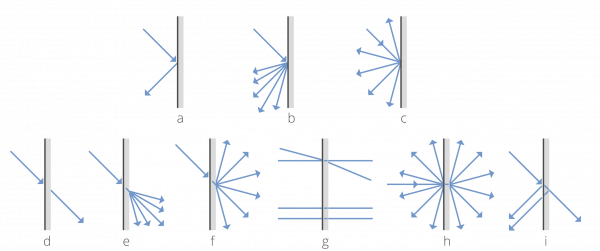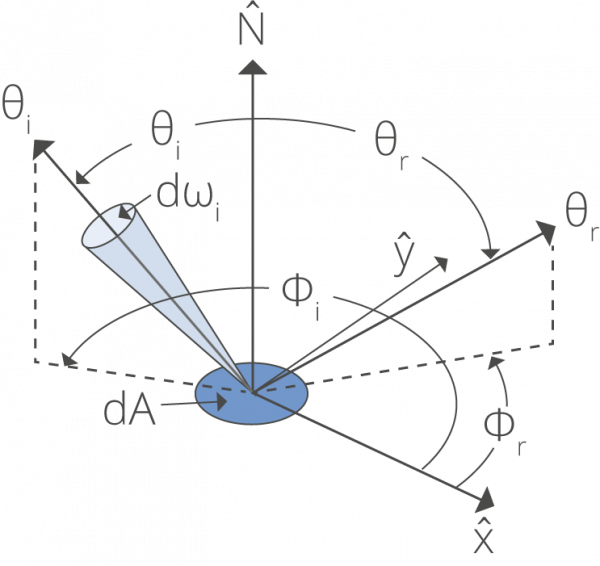#### Quick Contact

Phone: +49 (0)8193-93700-0
info@gigahertz-optik.de

Gigahertz-Optik, Inc. (US office)
Phone: +1-978-462-1818
info-us@gigahertz-optik.com

#### Watchlist

You can add products to the watchlist and compare them with one another or send us an inquiry. There are watchlist symbols on product pages and product tables for this purpose.

# 1.8 Reflection, Transmission and Absorption

Reflection is the process by which electromagnetic radiation is returned either at the boundary between two media (surface reflection) or at the interior of a medium (volume reflection), whereas transmission is the passage of electromagnetic radiation through a medium. Both processes can be accompanied by diffusion (also called scattering), which is the process of deflecting a unidirectional beam into many directions. In this case, we speak about diffuse reflection and diffuse transmission (Fig. 1). When no diffusion occurs, reflection or transmission of a unidirectional beam results in a unidirectional beam according to the laws of geometrical optics (Fig. 2). In this case, we speak about regular reflection (or specular reflection) and regular transmission (or direct transmission). Reflection, transmission and scattering leave the frequency of the radiation unchanged. Exception: The Doppler Effect causes a change in frequency when the reflecting material or surface is in motion.

Absorption is the transformation of radiant power to another type of energy, usually heat, by interaction with matter.Fig. 1: top: Direct, mixed and diffuse reflection bottom: direct, mixed and diffuse transmissionFig. 2: When directly reflected or directly transmitted, a unidirectional beam follows the laws of geometrical optics:
direct reflection (left): αin = αout, direct transmission (right): n1 × sin(αin) = n2 × sin(αout) with n1 and n2 denoting the respective medium’s index of refraction

### Reflectance ρ, Transmittance τ and Absorptance α

In general, reflection, transmission and absorption depend on the wavelength of the affected radiation. Thus, these three processes can either be quantified for monochromatic radiation (in this case, the adjective “spectral” is added to the respective quantity) or for a certain kind of polychromatic radiation. For the latter, the spectral distribution of the incident radiation has to be specified. In addition, reflectance, transmittance and absorptance might also depend on polarization and geometric distribution of the incident radiation, which therefore also have to be specified.

The reflectance ρ is defined by the ratio of reflected radiant power to incident radiant power. For a certain area element dA of the reflecting surface, the (differential) incident radiant power is given by the surface’s irradiance Ee multiplied with the size of the surface element, thus

e, incident = Ee dA

The (differential) reflected radiant power is given by the exitance Me multiplied with the size of the surface element:

e, reflected = Me dA

Thus,

 ρ = dΦe, reflected = Me × dA = Me dΦe, incident Ee × dA Ee

or

M= ρ Ee

Total reflectance is further subdivided in regular reflectance ρr and diffuse reflectance ρd, which are given by the ratios of regularly (or specularly) reflected radiant power and diffusely reflected radiant power to incident radiant power. From this definition, it is obvious that

ρ = ρr + ρd

The transmittance τ of a medium is defined by the ratio of transmitted radiant power to incident radiant power. Total transmittance is further subdivided in regular transmittance τr and diffuse transmittance τd, which are given by the ratios of regularly (or directly) transmitted radiant power and diffusely transmitted radiant power to incident radiant power.

Again,

τ = τr + τd

The absorptance α of a medium is defined by the ratio of absorbed radiant power to incident radiant power.

Being ratios of radiant power values, reflectance, transmittance and absorptance are dimensionless.

Quantities such as reflectance and transmittance are used to describe the optical properties of materials. The quantities can apply to complex radiation or monochromatic radiation. The optical properties of materials are not a constant since they are dependent on many parameters such as:

• thickness of the sample

• surface conditions

• angle of incidence

• temperature

• the spectral composition of the radiation (CIE standard illuminants A, B, C, D65 and other illuminants D)

• polarization effects

The measurement of optical properties of materials using integrating spheres is described in DIN 5036-3 and CIE 130-1998.

### Radiance coefficient qe, Bidirectional reflectance distribution function (BRDF)

The radiance coefficient qe characterizes the directional distribution of diffusely reflected radiation. In detail, the radiance coefficient depends on the direction of the reflected beam and is defined by the ratio of the radiance reflected in this direction to the total incident irradiance. In general, the reflected radiance is not independent from the directional distribution of the incident radiation, which therefore has to be specified.

In the USA, the concept of bidirectional reflectance distribution function BRDF is similar to the radiance coefficient. The only difference is that the BRDF is a function of the directions of the incident and the reflected beam (Fig. 3). In detail, the (differential) irradiance dEe impinging from a certain direction causes the reflected radiance dLe in another direction, which is given by

dLe = BRDF × dEe

This BRDF depends on more arguments than the radiance coefficient. However, its advantage is the simultaneous description of the material’s reflection properties for all possible directional distributions of incident radiation, whereas the radiance coefficient is generally valid for just one specific directional distribution of incident radiation.

The unit of radiance coefficient and BRDF is 1/steradian. The BRDF is often abbreviated as the Greek letter ρ, which can easily be confused with the reflectance (see previous paragraph Reflectance ρ, Transmittance τ and Absorptance α).Fig. 3: Geometry used to define the bidirectional reflectance distribution function (BRDF).
The BRDF depends on the directions of incident and reflected radiation.
These are given by the angles ϑi and ϑr (which are measured in relation to the reflecting surface’s normal)
and the azimuth angles φi and φr, (which are measured in the plane of the reflecting surface)

Source (valid as of 2002): http://math.nist.gov/~FHunt/appearance/brdf.html